Скачать презентацию Amortized Analysis Prof Muhammad Saeed Some of the

19961ebb9357aaf84e2cd01b5d297133.ppt

• Количество слайдов: 25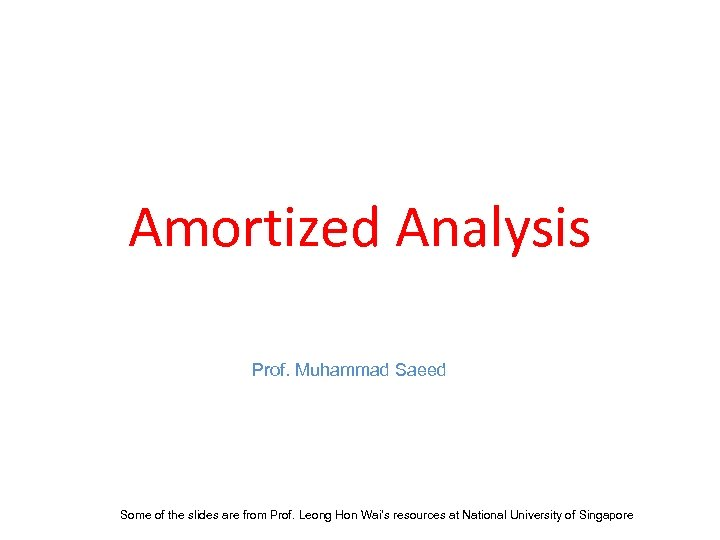Amortized Analysis Prof. Muhammad Saeed Some of the slides are from Prof. Leong Hon Wai’s resources at National University of Singapore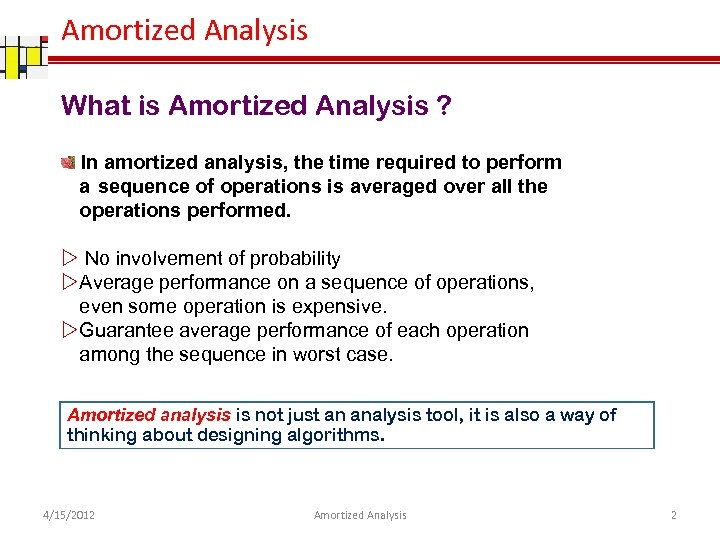Amortized Analysis What is Amortized Analysis ? In amortized analysis, the time required to perform a sequence of operations is averaged over all the operations performed. No involvement of probability Average performance on a sequence of operations, even some operation is expensive. Guarantee average performance of each operation among the sequence in worst case. Amortized analysis is not just an analysis tool, it is also a way of thinking about designing algorithms. 4/15/2012 Amortized Analysis 2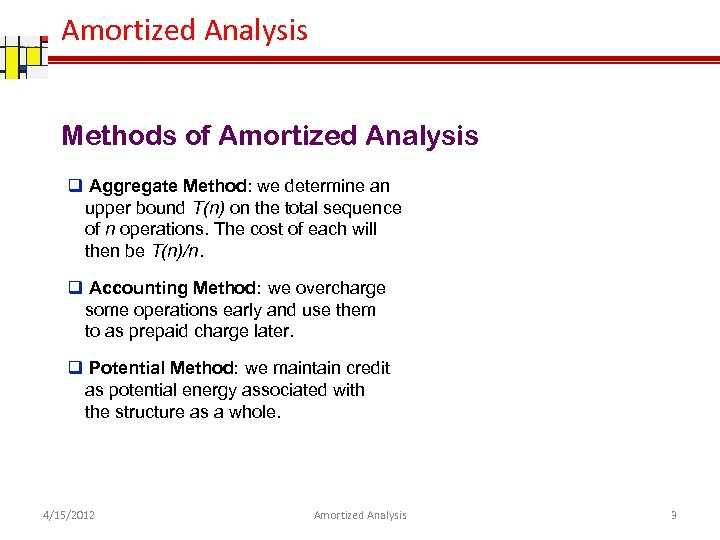Amortized Analysis Methods of Amortized Analysis q Aggregate Method: we determine an upper bound T(n) on the total sequence of n operations. The cost of each will then be T(n)/n. q Accounting Method: we overcharge some operations early and use them to as prepaid charge later. q Potential Method: we maintain credit as potential energy associated with the structure as a whole. 4/15/2012 Amortized Analysis 3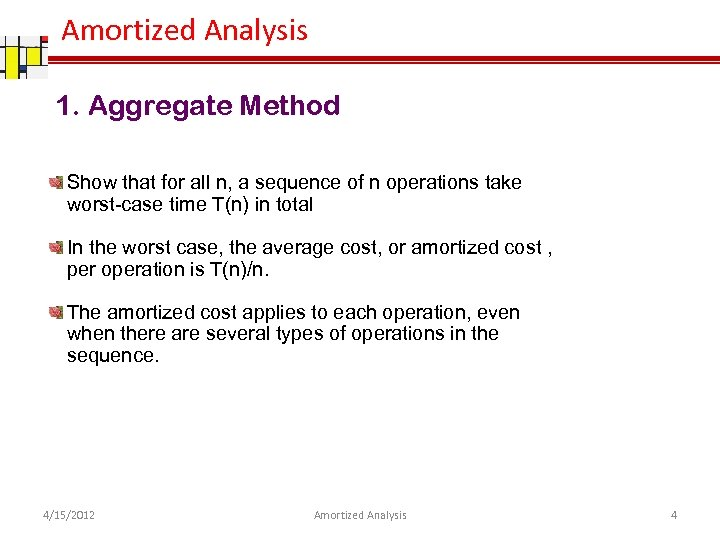Amortized Analysis 1. Aggregate Method Show that for all n, a sequence of n operations take worst-case time T(n) in total In the worst case, the average cost, or amortized cost , per operation is T(n)/n. The amortized cost applies to each operation, even when there are several types of operations in the sequence. 4/15/2012 Amortized Analysis 4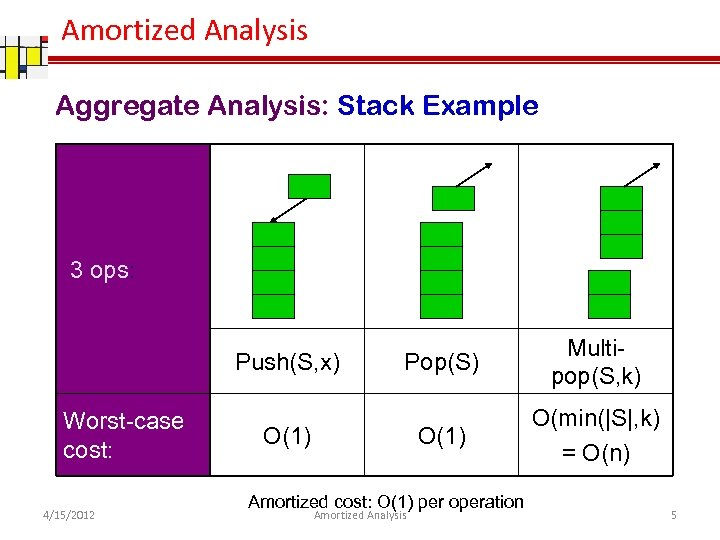Amortized Analysis Aggregate Analysis: Stack Example 3 ops: Worst-case cost: 4/15/2012 Pop(S) O(1) Push(S, x) Multipop(S, k) O(min(|S|, k) = O(n) O(1) Amortized cost: O(1) per operation Amortized Analysis 5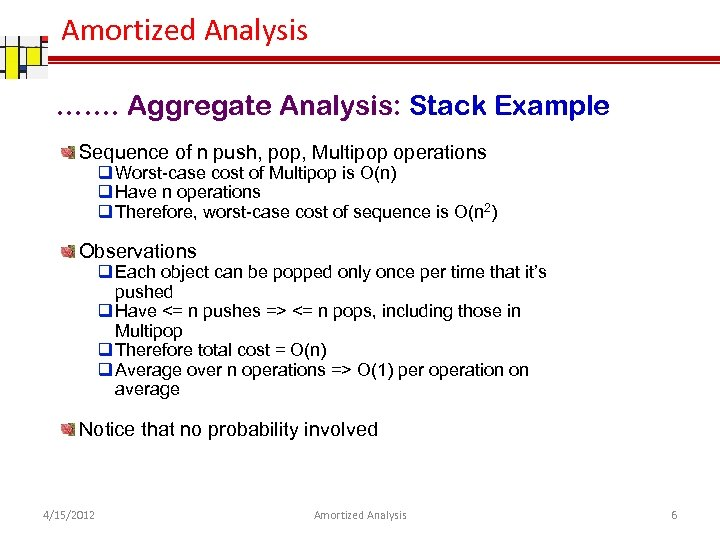Amortized Analysis ……. Aggregate Analysis: Stack Example Sequence of n push, pop, Multipop operations q Worst-case cost of Multipop is O(n) q Have n operations q Therefore, worst-case cost of sequence is O(n 2) Observations q Each object can be popped only once per time that it’s pushed q Have <= n pushes => <= n pops, including those in Multipop q Therefore total cost = O(n) q Average over n operations => O(1) per operation on average Notice that no probability involved 4/15/2012 Amortized Analysis 6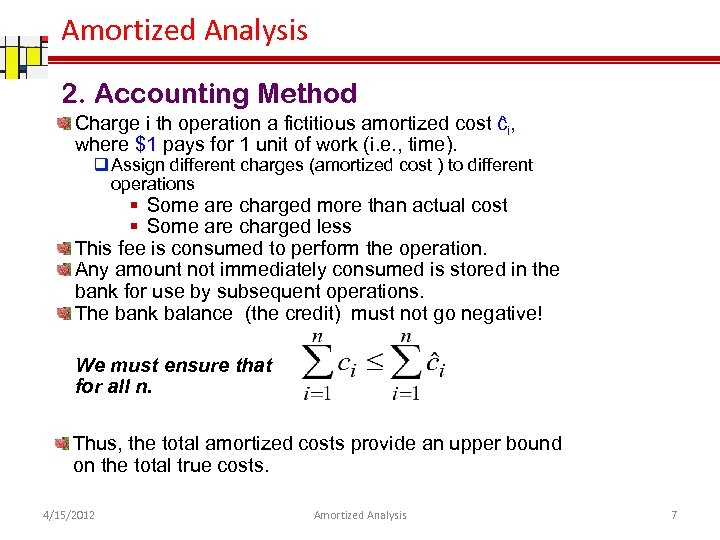Amortized Analysis 2. Accounting Method Charge i th operation a fictitious amortized cost ĉi, where \$1 pays for 1 unit of work (i. e. , time). q Assign different charges (amortized cost ) to different operations § Some are charged more than actual cost § Some are charged less This fee is consumed to perform the operation. Any amount not immediately consumed is stored in the bank for use by subsequent operations. The bank balance (the credit) must not go negative! We must ensure that for all n. Thus, the total amortized costs provide an upper bound on the total true costs. 4/15/2012 Amortized Analysis 7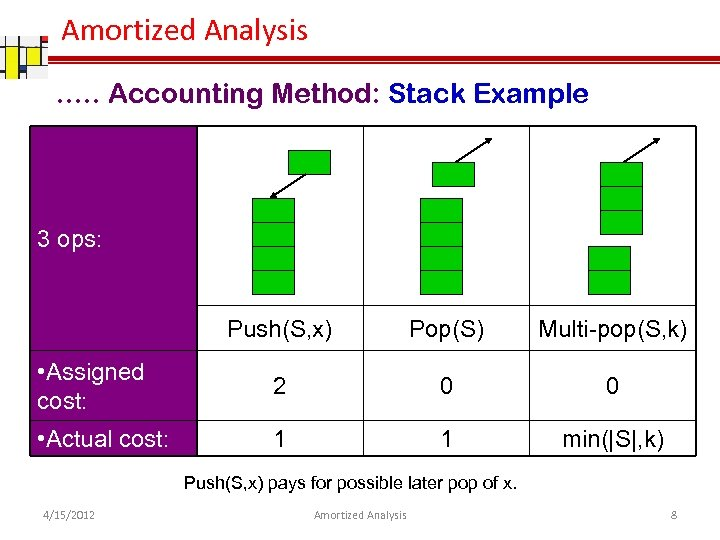Amortized Analysis …. . Accounting Method: Stack Example 3 ops: Push(S, x) Pop(S) Multi-pop(S, k) • Assigned cost: 2 0 0 • Actual cost: 1 1 min(|S|, k) Push(S, x) pays for possible later pop of x. 4/15/2012 Amortized Analysis 8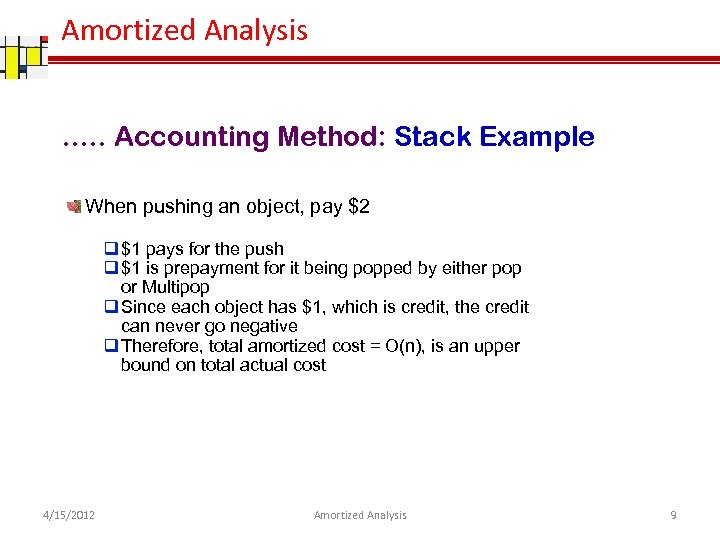Amortized Analysis …. . Accounting Method: Stack Example When pushing an object, pay \$2 q \$1 pays for the push q \$1 is prepayment for it being popped by either pop or Multipop q Since each object has \$1, which is credit, the credit can never go negative q Therefore, total amortized cost = O(n), is an upper bound on total actual cost 4/15/2012 Amortized Analysis 9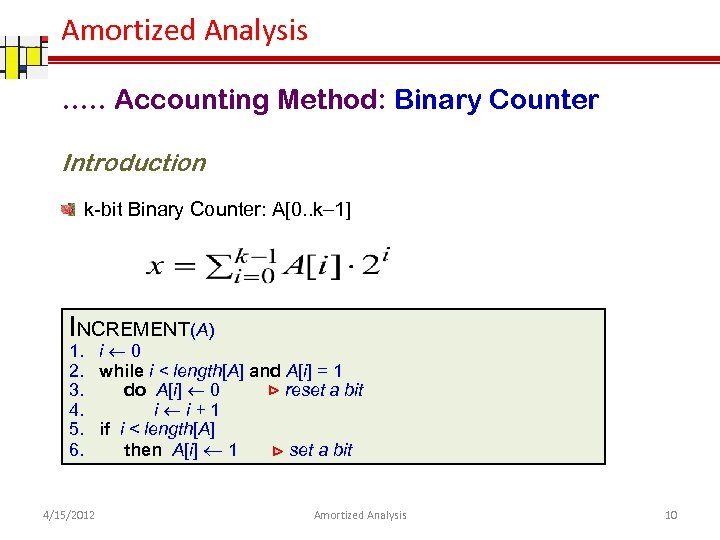Amortized Analysis …. . Accounting Method: Binary Counter Introduction k-bit Binary Counter: A[0. . k 1] INCREMENT(A) 1. i 0 2. while i < length[A] and A[i] = 1 3. do A[i] 0 ⊳ reset a bit 4. i i+1 5. if i < length[A] 6. then A[i] 1 ⊳ set a bit 4/15/2012 Amortized Analysis 10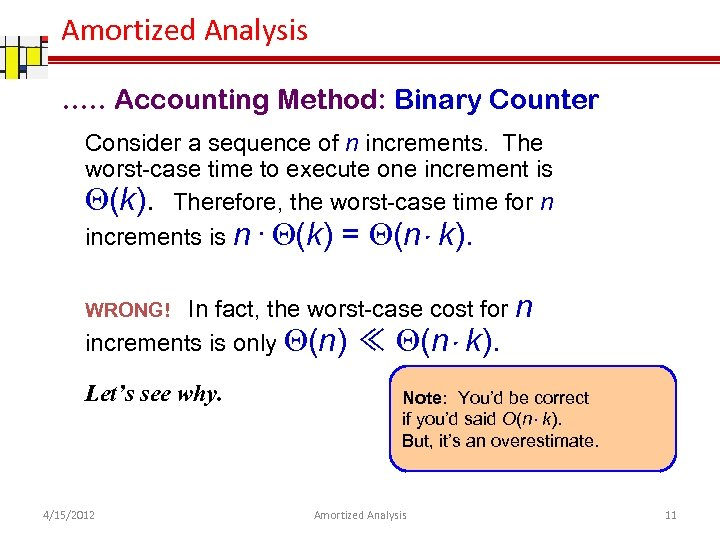Amortized Analysis …. . Accounting Method: Binary Counter Consider a sequence of n increments. The worst-case time to execute one increment is Q(k). Therefore, the worst-case time for n increments is n · Q(k) = Q(n k). In fact, the worst-case cost for n increments is only Q(n) ≪ Q(n k). WRONG! Let’s see why. 4/15/2012 Note: You’d be correct if you’d said O(n k). But, it’s an overestimate. Amortized Analysis 11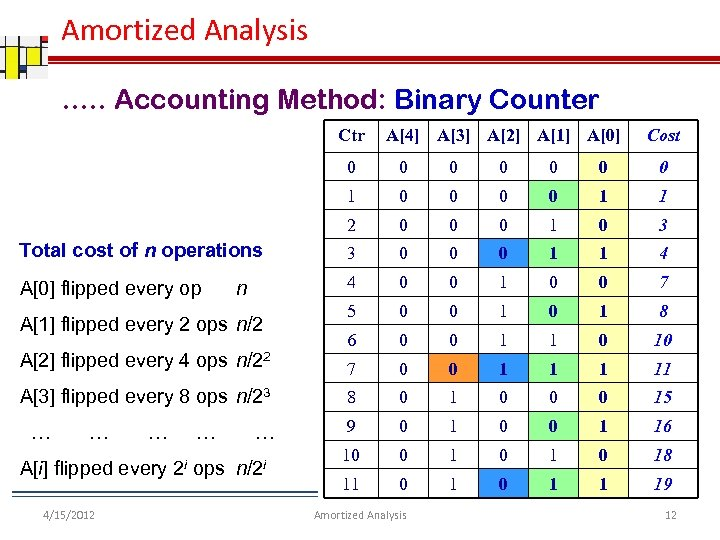Amortized Analysis …. . Accounting Method: Binary Counter Ctr A A A A A Cost 0 0 0 0 1 1 2 0 0 0 1 0 3 Total cost of n operations 3 0 0 0 1 1 4 A flipped every op 4 0 0 1 0 0 7 5 0 0 1 8 6 0 0 1 1 0 10 7 0 0 1 11 8 0 1 0 0 0 15 9 0 1 0 0 1 16 10 0 1 0 18 11 0 1 1 19 n A flipped every 2 ops n/2 A flipped every 4 ops n/22 A flipped every 8 ops n/23 … … … A[i] flipped every 2 i ops n/2 i 4/15/2012 Amortized Analysis 12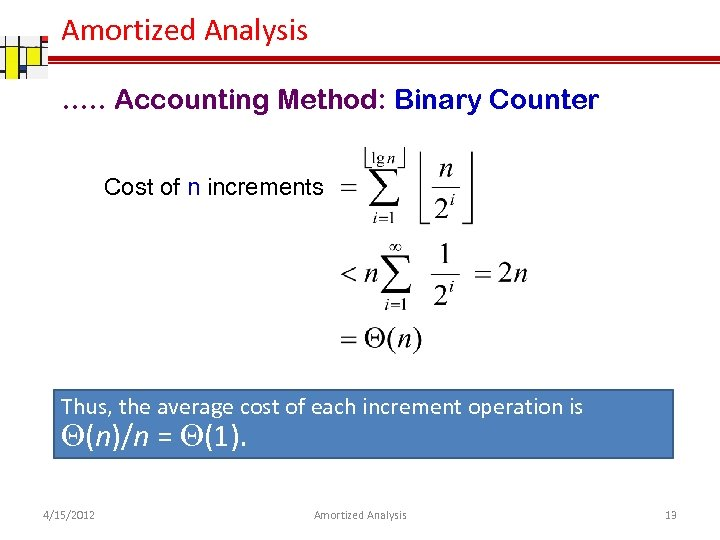Amortized Analysis …. . Accounting Method: Binary Counter Cost of n increments Thus, the average cost of each increment operation is Q(n)/n = Q(1). 4/15/2012 Amortized Analysis 13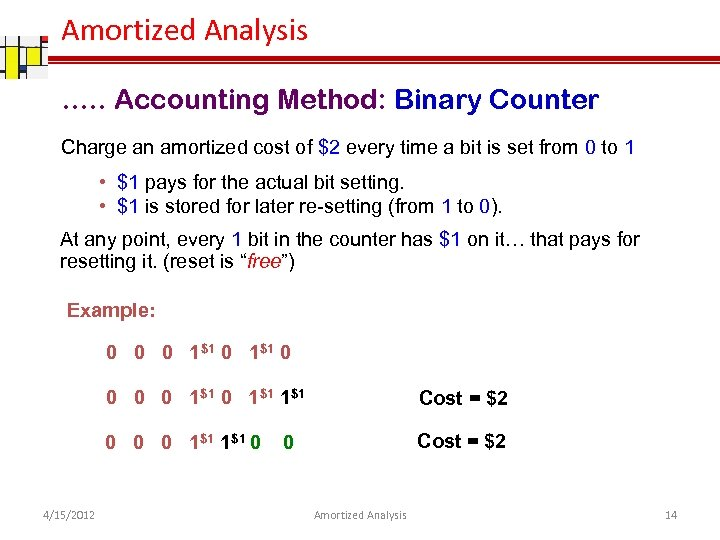Amortized Analysis …. . Accounting Method: Binary Counter Charge an amortized cost of \$2 every time a bit is set from 0 to 1 • \$1 pays for the actual bit setting. • \$1 is stored for later re-setting (from 1 to 0). At any point, every 1 bit in the counter has \$1 on it… that pays for resetting it. (reset is “free”) Example: 0 0 0 1\$1 0 1\$1 0 0 0 1\$1 0 4/15/2012 Cost = \$2 0 Amortized Analysis 14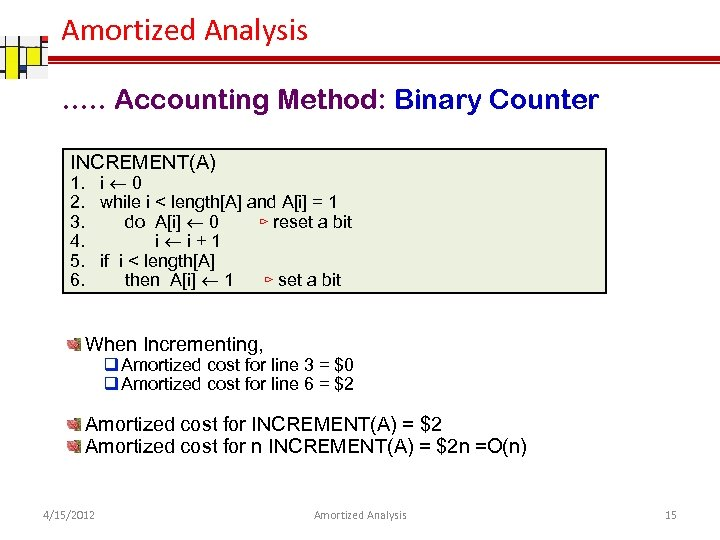Amortized Analysis …. . Accounting Method: Binary Counter INCREMENT(A) 1. i 0 2. while i < length[A] and A[i] = 1 3. do A[i] 0 ⊳ reset a bit 4. i i+1 5. if i < length[A] 6. then A[i] 1 ⊳ set a bit When Incrementing, q Amortized cost for line 3 = \$0 q Amortized cost for line 6 = \$2 Amortized cost for INCREMENT(A) = \$2 Amortized cost for n INCREMENT(A) = \$2 n =O(n) 4/15/2012 Amortized Analysis 15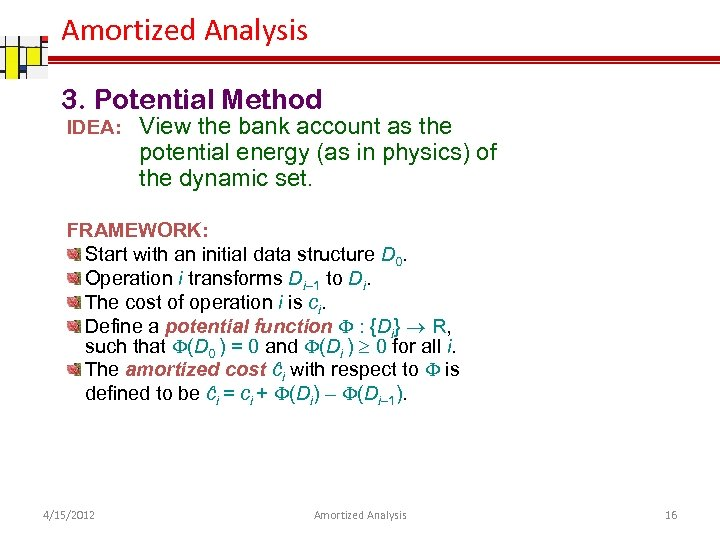Amortized Analysis 3. Potential Method IDEA: View the bank account as the potential energy (as in physics) of the dynamic set. FRAMEWORK: Start with an initial data structure D 0. Operation i transforms Di– 1 to Di. The cost of operation i is ci. Define a potential function F : {Di} R, such that F(D 0 ) = 0 and F(Di ) ³ 0 for all i. The amortized cost ĉi with respect to F is defined to be ĉi = ci + F(Di) – F(Di– 1). 4/15/2012 Amortized Analysis 16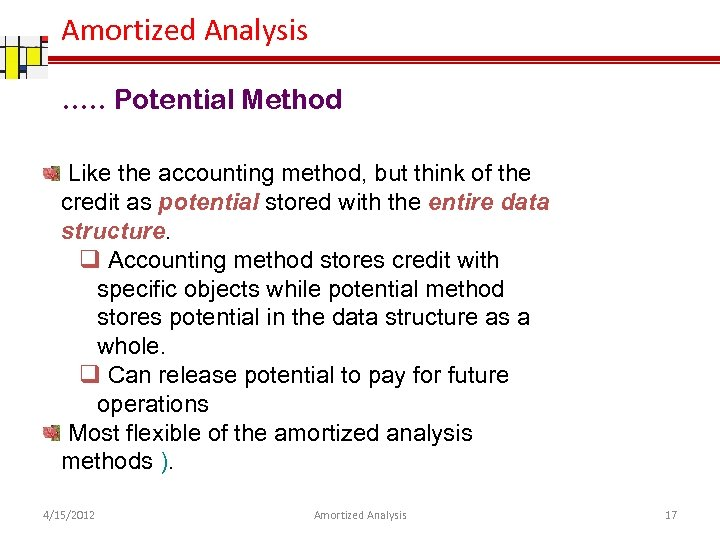Amortized Analysis …. . Potential Method Like the accounting method, but think of the credit as potential stored with the entire data structure. q Accounting method stores credit with specific objects while potential method stores potential in the data structure as a whole. q Can release potential to pay for future operations Most flexible of the amortized analysis methods ). 4/15/2012 Amortized Analysis 17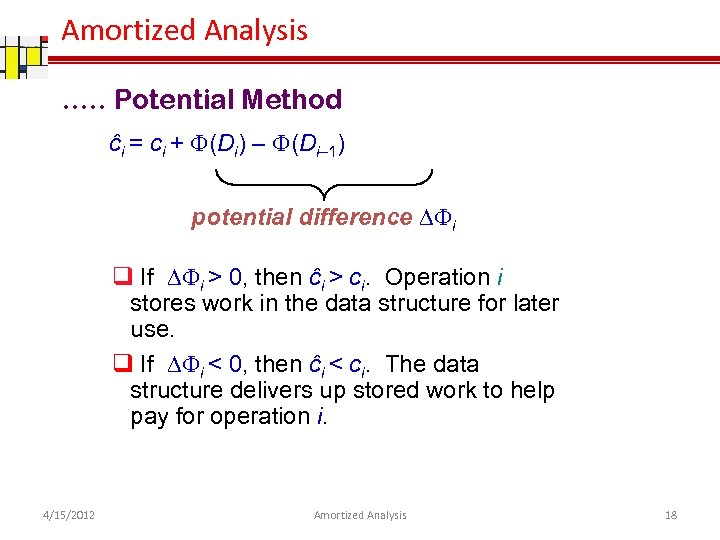Amortized Analysis …. . Potential Method ĉi = ci + F(Di) – F(Di– 1) potential difference DFi q If DFi > 0, then ĉi > ci. Operation i stores work in the data structure for later use. q If DFi < 0, then ĉi < ci. The data structure delivers up stored work to help pay for operation i. 4/15/2012 Amortized Analysis 18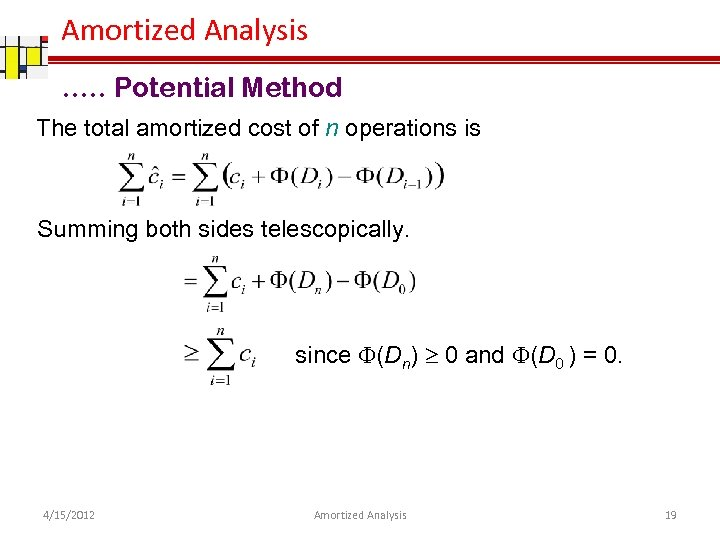Amortized Analysis …. . Potential Method The total amortized cost of n operations is Summing both sides telescopically. since F(Dn) ³ 0 and F(D 0 ) = 0. 4/15/2012 Amortized Analysis 19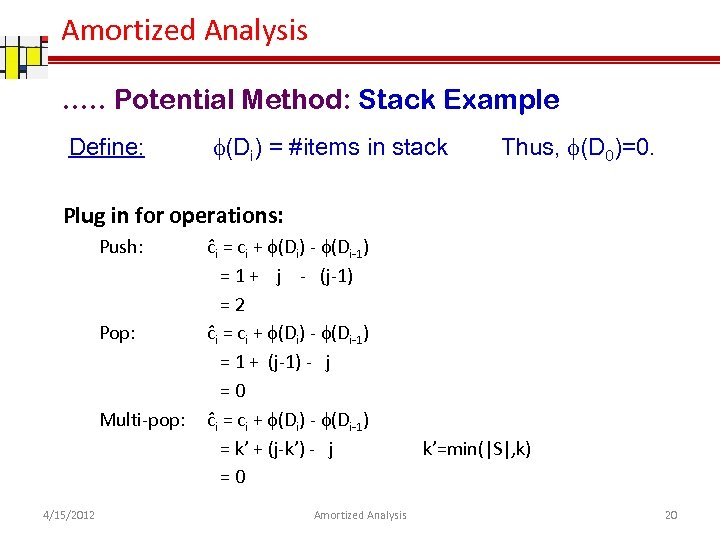Amortized Analysis …. . Potential Method: Stack Example Define: (Di) = #items in stack Thus, (D 0)=0. Plug in for operations: Push: Pop: Multi-pop: 4/15/2012 ĉi = ci + (Di) - (Di-1) = 1 + j - (j-1) =2 ĉi = ci + (Di) - (Di-1) = 1 + (j-1) - j =0 ĉi = ci + (Di) - (Di-1) = k’ + (j-k’) - j =0 Amortized Analysis k’=min(|S|, k) 20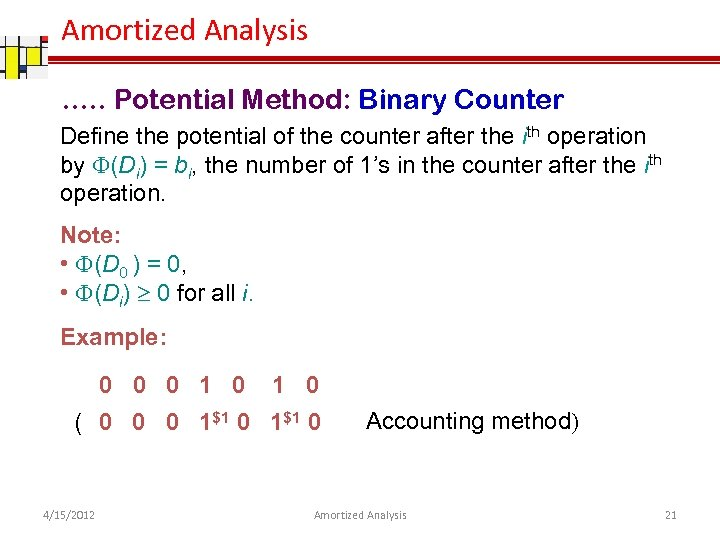Amortized Analysis …. . Potential Method: Binary Counter Define the potential of the counter after the ith operation by F(Di) = bi, the number of 1’s in the counter after the ith operation. Note: • F(D 0 ) = 0, • F(Di) ³ 0 for all i. Example: 0 0 0 1 0 ( 0 0 0 1\$1 0 4/15/2012 Accounting method) Amortized Analysis 21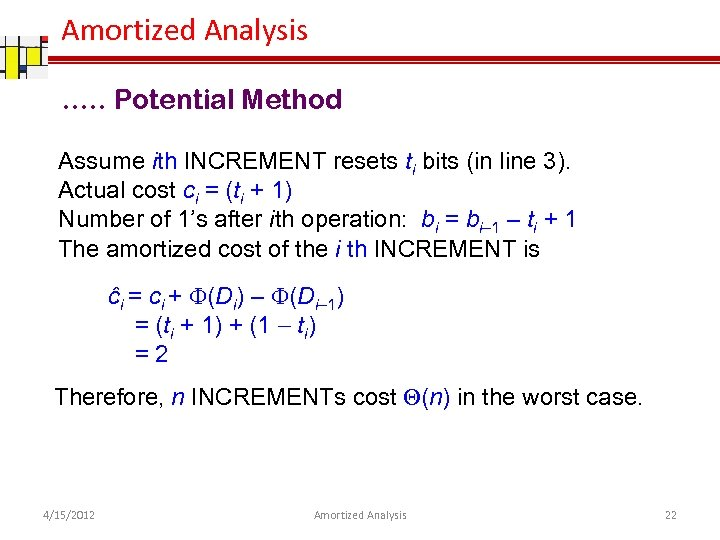Amortized Analysis …. . Potential Method Assume ith INCREMENT resets ti bits (in line 3). Actual cost ci = (ti + 1) Number of 1’s after ith operation: bi = bi– 1 – ti + 1 The amortized cost of the i th INCREMENT is ĉi = ci + F(Di) – F(Di– 1) = (ti + 1) + (1 ti) =2 Therefore, n INCREMENTs cost Q(n) in the worst case. 4/15/2012 Amortized Analysis 22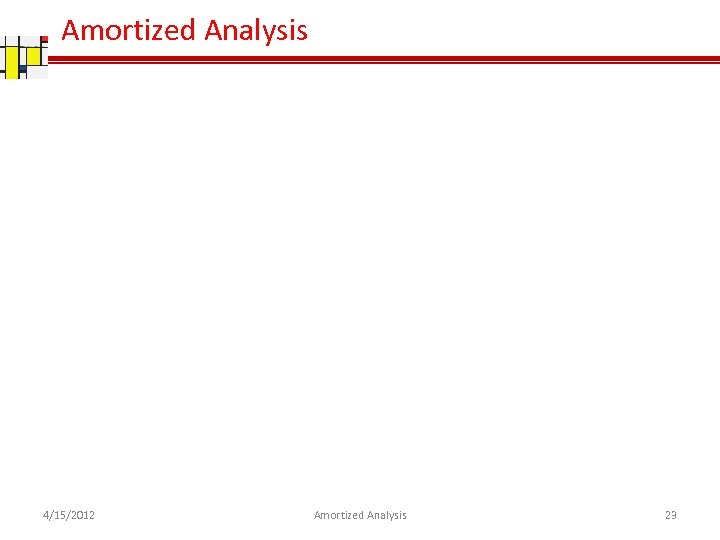Amortized Analysis 4/15/2012 Amortized Analysis 23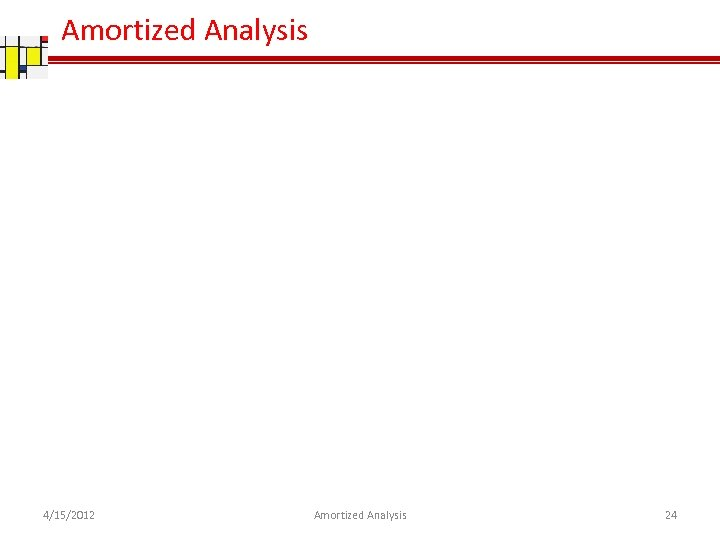Amortized Analysis 4/15/2012 Amortized Analysis 24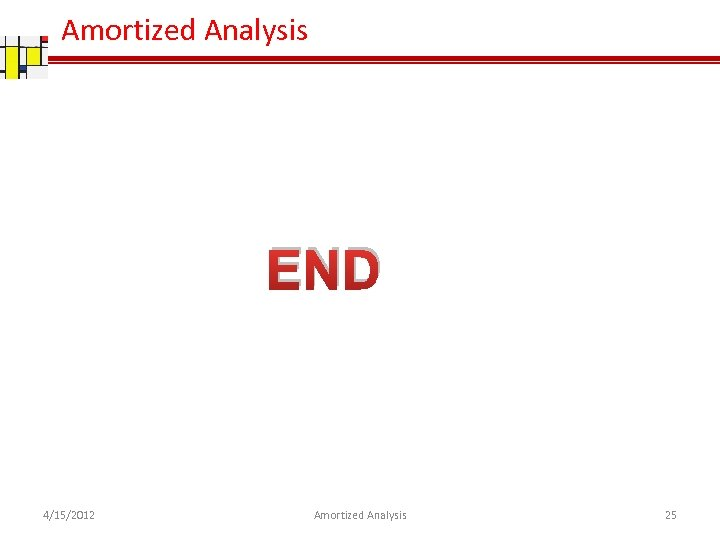Amortized Analysis END 4/15/2012 Amortized Analysis 25